# Difference between revisions of "Jacobson category"

An abstract model of the category of Jacobson-commutative algebras (cf. Commutative algebra). It is a special instance of a Zariski category. To be precise, it a Zariski category in which all objects are Jacobson objects, i.e., objects whose prime congruences are meets of maximal congruences. Jacobson categories are axiomatically defined as categories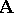satisfying the following five axioms:

1)is co-complete, i.e., has all small colimits;

2)has a strong generating set of objects whose objects are finitely presentable and directly co-disjunctable;

3) regular epimorphisms inare universal, i.e., stable under pull-backs along any morphism (cf. also Morphism);

4) the terminal object ofis finitely presentable and has no proper subobject;

5) the product of two objects inis co-universal, i.e., stable under pushouts along any morphism.

The notion of a directly co-disjunctable object used in 2) is defined as follows. A morphisminis a direct factor morphism if there is a morphism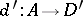insuch that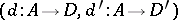is a product of objects in. An object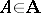is directly co-disjunctable if the pair of inductions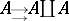ofinto its coproduct by itself has a co-disjunctor (cf. Zariski category) that is a direct factor morphism.

What makes Jacobson categories special is the fact that co-disjunctors are co-equalizers or, more concretely, that algebras of fractions are quotient algebras. It follows that integral or local reduced algebras are fields, that reduced algebras are von Neumann regular (cf. also Regular ring (in the sense of von Neumann)), that prime ideals are maximal, that prime spectra are Hausdorff and Boolean spaces, that the set of finitely generated ideals of an algebra is equipped with, next to the usual addition and multiplication of ideals, an extra multiplication of ideals, giving it the structure of a Stone semi-algebra, etc.

A typical example of a Jacobson category is the categoryof algebraic commutative algebras over a commutative ring(cf. also Algebraic algebra), i.e., algebras whose elements are algebraic over. More generally, the category of algebraic objects of any Zariski categoryis a Jacobson category, and is indeed the universal Jacobson category associated to[a2].

How to Cite This Entry:
Jacobson category. Encyclopedia of Mathematics. URL: http://encyclopediaofmath.org/index.php?title=Jacobson_category&oldid=12131
This article was adapted from an original article by Y. Diers (originator), which appeared in Encyclopedia of Mathematics - ISBN 1402006098. See original article